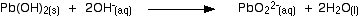# Question #370a7

Jun 26, 2015

$P {b}_{\left(a q\right)}^{2 +} + 2 O {H}_{\left(a q\right)}^{-} \rightarrow P b {\left(O H\right)}_{2 \left(s\right)}$

#### Explanation:

By $N {H}_{3}$ I am assuming you mean ammonia solution.

This partly ionises in water to give ammonium and hydroxide ions:

$N {H}_{3 \left(g\right)} + {H}_{2} {O}_{\left(l\right)} r i g h t \le f t h a r p \infty n s N {H}_{4 \left(a q\right)}^{+} + O {H}_{\left(a q\right)}^{-}$

$P {b}_{\left(a q\right)}^{2 +} + 2 O {H}_{\left(a q\right)}^{-} \rightarrow P b {\left(O H\right)}_{2 \left(s\right)}$

The same reaction happens with $N a O H$ solution.

The sodium ions remain in solution as spectator ions.

If XS sodium hydroxide is added the precipitate redissolves to give the soluble plumbate(II) ion.

A simple way of writing this is:(chemguideUK)

Ammonia solution can't do this as the concentration of $O {H}^{-}$ ions is not high enough.